﻿ Math Simple Equation On Chalk Board. One Plus One Equals Two Stock Photo - Image of white, board: 122029134

# Math simple equation on chalk board. One plus One equals two.

## Math simple equation on chalk board. One plus One equals two.

More similar stock images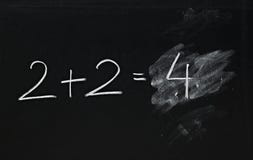Math simple equation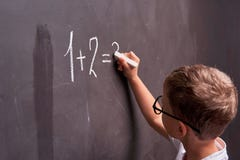Primary education. Rear view of a schoolboy solves a mathematical example on a blackboard in a math class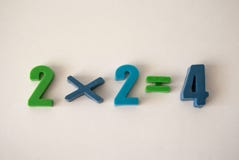School numbers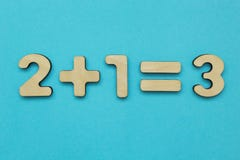A simple mathematical example for children on a blue background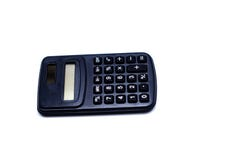Equipment for calculating numbers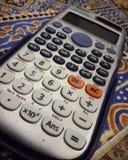Calculator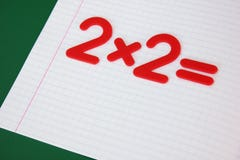A simple mathematical example in a clean school notebook. Back to school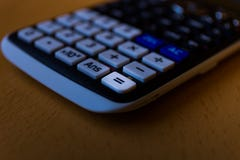Equal key of a scientific calculator keyboard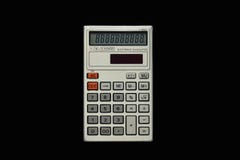Used simple pocket calculator on isolated bkack background top view.
More stock photos from Olga Vorobeva's portfolio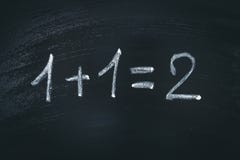Math simple equation on chalk board. One plus One equals two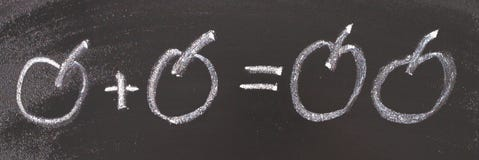Math simple equation on chalk board. One plus One equals two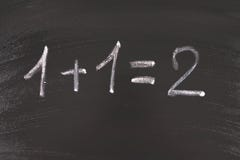Math simple equation on chalk board. One plus One equals two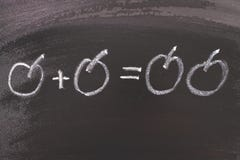Math simple equation on chalk board. One plus One equals two